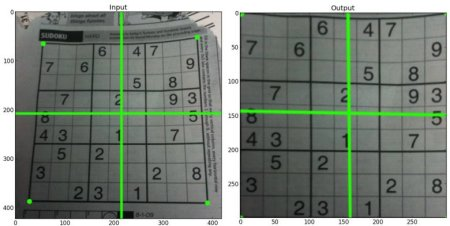# OpenCV-Python 系列 十二 | 图像的几何变换

### 目标

• 学习将不同的几何变换应用到图像上，如平移、旋转、仿射变换等。
• 你会看到这些函数: cv.getPerspectiveTransform

### 变换

OpenCV提供了两个转换函数cv.warpAffinecv.warpPerspective，您可以使用它们进行各种转换。cv.warpAffine采用2×3转换矩阵，而cv.warpPerspective采用3×3转换矩阵作为输入。

#### 缩放

import numpy as np
import cv2 as cv
res = cv.resize(img,None,fx=2, fy=2, interpolation = cv.INTER_CUBIC)
#或者
height, width = img.shape[:2]
res = cv.resize(img,(2*width, 2*height), interpolation = cv.INTER_CUBIC)


#### 平移

M = begin{bmatrix} 1&0&t_x 0&1&t_y end{bmatrix}

import numpy as np
import cv2 as cv
rows,cols = img.shape
M = np.float32([[1,0,100],[0,1,50]])
dst = cv.warpAffine(img,M,(cols,rows))
cv.imshow('img',dst)
cv.waitKey(0)
cv.destroyAllWindows()


cv.warpAffine函数的第三个参数是输出图像的大小，其形式应为(width，height)。记住width =列数，height =行数。#### 旋转

M = begin{bmatrix} costheta&-sintheta sintheta&costheta end{bmatrix}

begin{bmatrix} alpha&beta&(1- alpha ) cdot center.x – beta cdot center.y – beta&alpha&beta cdot center.x + (1- alpha ) cdot center.y end{bmatrix}

begin{array}{l} alpha = scale cdot cos theta , beta = scale cdot sin theta end{array}

img = cv.imread('messi5.jpg',0)
rows,cols = img.shape
# cols-1 和 rows-1 是坐标限制
M = cv.getRotationMatrix2D(((cols-1)/2.0,(rows-1)/2.0),90,1)
dst = cv.warpAffine(img,M,(cols,rows))#### 仿射变换

img = cv.imread('drawing.png')
rows,cols,ch = img.shape
pts1 = np.float32([[50,50],[200,50],[50,200]])
pts2 = np.float32([[10,100],[200,50],[100,250]])
M = cv.getAffineTransform(pts1,pts2)
dst = cv.warpAffine(img,M,(cols,rows))
plt.subplot(121),plt.imshow(img),plt.title('Input')
plt.subplot(122),plt.imshow(dst),plt.title('Output')#### 透视变换

img = cv.imread('sudoku.png')
rows,cols,ch = img.shape
pts1 = np.float32([[56,65],[368,52],[28,387],[389,390]])
pts2 = np.float32([[0,0],[300,0],[0,300],[300,300]])
M = cv.getPerspectiveTransform(pts1,pts2)
dst = cv.warpPerspective(img,M,(300,300))
plt.subplot(121),plt.imshow(img),plt.title('Input')
plt.subplot(122),plt.imshow(dst),plt.title('Output')
plt.show()### 其他资源

1. “Computer Vision: Algorithms and Applications”, Richard Szeliski